## Mathematics Paper 2 Questions and Answers - Baringo North Joint Evaluation Mock Exams 2022

Instructions to candidates

• The paper contains two sections: Section I and Section II.
• Answer all the questions in Section I and only five questions from Section II
• All answers and working must be written on the question paper in the spaces provided below each question.
• Show all the steps in your calculations, giving your answers at each stage in the spaces below each question.
• Non – programmable silent electronic calculators and KNEC Mathematical tables may be used, except where stated otherwise.

SECTION 1 (50 MARKS)
Answer all questions in this section.

1. Solve for x in the equation 2Sin2x - 1 = Cos2x + Sinx for 0°≤x≤360°(3marks)
2.
1. Expand (1+3/x)5 up to the fifth term (2marks)
2. Hence use your expansion to evaluate the value of (2.5)5 to 3 d.p. (2 marks)
3. Complete the table below for y = 8 − 2x − x2 for −4 ≤ x ≤ 2.
 x −4 −3 −2 −1 0 1 2 y
Hence use trapezium rule with six strips to find the area of the region bounded by the curve and the x- axis. (3marks)
4. Make p the subject of the formula (3 marks)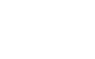5. The figure below shows a rectangular based right pyramid. Find the angle between the planes ABCD and ABV. (3marks)6. An object A of area 10cm2 is mapped onto its image B of area 60cm2 by a transformation whose matrix is given by P=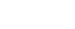. Find the possible values of x. (3 marks)
7. Find the value of x in the equation        (3marks)
log105 − 2 + log10(2x + 10) = log10(x − 4)
8. The data below shows marks obtained by 10 students in a test.
71, 55, 69, 45, 65, 57, 71, 82, 55, 50. Calculate the standard deviation using an assumed mean of 60. (3marks)
9. Evaluate by rationalizing the denominator and leaving your answer in surd form. (3marks)
√8
1 + Cos45°
10. The position vectors fof points A and B are 5i + 4− 6k and 2i − 2j respectively. A point X divides AB in the ratio −3: 5. Find the coordinates of X. (3marks)
11. A closed box has a square base of side x metres and its height h metres. The total surface area of the box is 24m2.
1. Find the expression of h in terms of x.
2. Hence find the value of x that would make the volume of the box maximum. (4 marks)
12. M varies directly as D and as the cube of V. Calculate the percentage change in M when V is increased by 10% and D is reduced by 10%. (3marks)
13. Find the value of t if the gradient of the graphs of the functions y = x2 − x3 and y = x − tx2 are equal at  x = 1/3 . (3marks)
14. The image of a point A, under the transformation represented by the matrix T =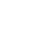is A’ (-2, 4)Find the coordinates of A (3marks)
15. In the figure below, ABC is a tangent at B and CDE is a straight line. ∠BED=50°, ∠DEF=35° and ∠ECB=25°.Calculate the values of x and z. (2marks)
16. The equation of a circle is given by 4x2 + 4y2 − 8x + 2y − 7 = 0
Determine the coordinates of the centre of the circle. (3marks)

SECTION II (50 MARKS)
Answer only five questions from this section

1.
1. Using a ruler and a pair of compasses only construct triangle ABC in whichAB = 6cm, BC = 5.5cm and angle ABC = 60°. Measure AC. (3marks)
2. On the same side of AB as C, determine the locus of a point P such that angle APB = 60° (2marks)
3. Construct the locus of R such that AR = 3cm (1mark)
4. Identify the region T such that AR≥ 3cm and angleAPB≥ 60° by shading the unwanted part. (2marks)
5. Determine point Q such that area of AQB is half the area of ABC and that Angle AQB = 60°. (2marks)
2. A sequence is formed by adding corresponding terms of an AP and GP. The first, second and third terms of the sequence formed are 14, 34 and 78 respectively.
1. Given that the common ratio of the GP is 3;
1. Find the first term of the AP and GP and the common difference of the AP. (2marks)
2. Find the sixth term and the sum of the first six terms of the sequence. (3marks)
2. The second and third terms of a geometric progression are 24 and 12(x + 1) respectively.
Find the whole number value of x and hence the first term given the sum of the first three terms of the progression is 76. (5marks)
3. Income tax rate are as shown below.
 Income (k£ p.a) Rate (Ksh per £) 1- 4200 2 4201 - 8000 3 8001 - 12600 5 12601 – 16800 6 16801 and above 7
Omari pays Sh. 4000 as P.A.Y.E per month. He has a monthly house allowance of Ksh. 10800 and is entitled to a personal relief of Ksh. 1,100 per month. Determine;
1. his gross tax p.a in Ksh (2marks)
2. his taxable income in k£ p.a (4marks)
3. his basic salary in Ksh. p.m (2marks)
4. his net salary per month (2marks)
4. The diagram below shows a sketch of the line y = 3x and the curve y = 4 − x2 intersecting at point P and Q.1. Find the co-ordinates of P and Q (4marks)
2. Given that QN is perpendicular to the x-axis at N, calculate
1. the area bounded by the curve y = 4 − x2 , the x-axis and line QN. (2marks)
2. the area of the shaded region that lies below the x - axis (2marks)
3. the area of the region enclosed by the curve y = 4 − x2, the line y = 3x and the y-axis (2marks)
5. The positions of two towns A and B are (500N, 450W) and (500N, K0W) respectively. It takes a plane 5 hours to travel from A to B at an average speed of 800knots. The same plane takes 1½ hours to travel from B to another town C at the same average speed. Given that C is to the north of B, calculate to the nearest degree,
1. The value of K (4marks)
2. The latitude of C (3marks)
3. If the plane started from A at 9.00am and flew to C through B, find the local time at C when the plane arrived there. (3marks)
6.
1. Complete the table below for the equation y = x3 + 2x2 to 2 d.p(2marks)
 x −3 −2.5 −2 −1.5 −1 −0.5 0 0.5 1 1.5 2x2 18 12.5 8 4.5 2 0 1 4.5 x3 −27 −8 −1 0 1 y −9 0 1 0 2
2. On the grid provided, draw the graph of y = x3 + 2x2 for −3 ≤ x ≤ 1.5. Take a scale of 2cm to represent 1 unit on the x- axis and 1cm to represent 1unit on the y – axis. (3marks)
3. Use your graph to solve
1. x3 + 2x2 = 0        (2marks)
2. x3 + 2x2 − x − 2 = 0      (3marks)
7. The table below shows the marks obtained by 47 students in a mathematics test.
 Marks 31 – 35 36 – 40 41 – 45 46 – 50 51 – 55 56 - 60 No. of candidates 4 6 12 15 8 2
1. On the grid provided, draw a cumulative frequency curve. (3marks)
1. The median (2marks)
2. The semi interquartile range (2marks)
2. In order to pass the test a candidate had to score more than 40 marks. Calculate the percentage of candidates who passed. (3marks)
8. In the triangle OPQ below, OP = p and OQ = q. R is a point on PQ such that PR: RQ = 1 : 3 and 5OS = 2 OQ. PS intersects OR at T.1. Express in term of p and q
1. OS (1mark)
2. PQ (1mark)
3. OR (2mark)
2. Given that OT= hOR and PT = kPS. Determine the values of h and k. (6marks)## MARKING SCHEME

SECTION 1 (50 MARKS)
Answer all questions in this section.

1. Solve for x in the equation 2Sin2x - 1 = Cos2x + Sinx for 0°≤x≤360°(3marks)
2Sin2x − 1 = 1 − Sin2x + sin x
2Sin2x + Sin2x − sinx −1 − 1 =0
3sin2x − sin x − 2 = 0
3sin2x − 3 sinx + 2sinx − 2 = 0
3Sinx(Sinx − 1) + 2 (Sinx − 1) = 0
(Sinx − 1) (3Sinx + 2) = 0
Sinx − 1 = 0
Sin x = 1
x = 90°
OR
3Sinx−2
3         3
Sin x = −2/3
x = 180 + 41.81 = 138.19
x = 360 − 41.81 = 318.19
2.
1. Expand (1+3/x)5 up to the fifth term (2marks)
1  5  10  10  5
1 + 5(3/x) + 10(3/x)2 + 10(3/x)3 + 5(3/x)4
1 + 15 + 90 + 270 + 405
x      x2      x3       x4
2. Hence use your expansion to evaluate the value of (2.5)5 to 3 d.p. (2 marks)
2.5 = 1 + 3/x
2.5 − 1 = 3/x
1.5 = 3/x
1.5 3
1.5    1.5
x = 2
1 + 15 + 90 + 270 + 405
2      4       8       16
= 90.0625
= 90.063
3. Complete the table below for y = 8 − 2x − x2 for −4 ≤ x ≤ 2.
 x −4 −3 −2 −1 0 1 2 y 0 5 8 9 8 5 0
Hence use trapezium rule with six strips to find the area of the region bounded by the curve and the x- axis. (3marks)
A = ½ × 1 {0+0+2(5+8+9+8+5)}
½ × 2(35)
= 35
4. Make p the subject of the formula (3 marks)p − 3u
1       y − 3xp
e²(y −3xp) = p − 3u
e²y −3e²xp = p − 3u
e²y + 3u = p + 3e²xp
p + 3e²xp = e²y + 3u
p(1 + 3e2x) = e2y + 3u
p = e2y + 3u
1 + 3e2x
5. The figure below shows a rectangular based right pyramid. Find the angle between the planes ABCD and ABV. (3marks)VM = √(82 − 22)
= √60 = 7.746CosM =   1.5
7.746
Cos M = 0.19365
Cos−1  0.19365
= 78.83°
6. An object A of area 10cm2 is mapped onto its image B of area 60cm2 by a transformation whose matrix is given by P=. Find the possible values of x. (3 marks)
Area  scale factor = 60/10 = 6Determinant
x(x + 3) − 12 = x2 + 3x − 12
x2 + 3x − 12 = 6
x2 + 3x − 12 − 6 = 0
x2 + 3x − 18 = 0
x2 + 6x − 3x − 18 = 0
x(x + 6) − 3(x + 6) = 0
(x + 6) (x−3) = 0
x + 6 = 0   x − 3 = 0
x = −6            x = 3
7. Find the value of x in the equation        (3marks)
log105 − 2 + log10(2x + 10) = log10(x − 4)
log105 − 2log1010 + log10(2x + 10) = log10(x − 4)
log105(2x + 10) − log10100 = log10(x − 4)
log10(10x + 50) = log10(x − 4)
100
10x + 50 = x − 4
100           1
10x + 50 = 100(x − 4)
10x + 50 = 100x − 400
50 + 400 = 100x − 10x
450 = 90x
90     90
x = 5
8. The data below shows marks obtained by 10 students in a test.
71, 55, 69, 45, 65, 57, 71, 82, 55, 50. Calculate the standard deviation using an assumed mean of 60. (3marks)= √(121.6 − 4)
= √117.6
= 10.84
9. Evaluate by rationalizing the denominator and leaving your answer in surd form. (3marks)
√8
1 + Cos45°
(√8) √2
(1 + 1/√2)√2
√16  =   4
√2+1   √2+1
4    × √2 − 1
√2+1    √2 − 1
4√2− 4
2 − 1
= 4√2 − 4
10. The position vectors fof points A and B are 5i + 4− 6k and 2i − 2j respectively. A point X divides AB in the ratio −3: 5. Find the coordinates of X. (3marks)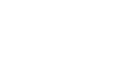X ( 9.5, 13, −15)
11. A closed box has a square base of side x metres and its height h metres. The total surface area of the box is 24m2.
1. Find the expression of h in terms of x.S.A = 2x2 + 4xh
2x2 + 4xh = 24
4xh = 24 − 2x2
h = 24 − 2x2
4x
h = 24 − 2x²
4x     4x
h = 6/x − x/2
2. Hence find the value of x that would make the volume of the box maximum. (4 marks)
V = b.a × h
= x× h
=hx2
V = hx2
x2 = 6/x − x/2
V = 6x −
2
dv/dx = 6 3x2
2
3x2  = 0
2
6 = 3x2
2
3x2  = 0
2
12 = 3x2
3      2
√4 = √x2
2 = x

12. M varies directly as D and as the cube of V. Calculate the percentage change in M when V is increased by 10% and D is reduced by 10%. (3marks)
M = KDV3
M1 = K(0.9D) (1.1V)3
M1 = 0.9 × 1.13KDV3
M1 = 1.1979KDV3
= 1.1979M
%Change = 1.1979M − M × 100
M
= 0.1979 × 100
= 19.79%
13. Find the value of t if the gradient of the graphs of the functions y = x2 − x3 and y = x − tx2 are equal at  x = 1/3 . (3marks)
y = x2 − x3
dy/dx = 2x − 3x2
y = x − tx2
dy/dx = 1 − 2tx
x = 1/3
dy/dx = 2(1/3) − 3(1/3)2
= 2/3 − 1/3 = 1/3
dy/dx = 1 − 2t(1/3)
= 1 − 2/3t
1/3 = 1 − 2/3t
2/3t = 1 − 1/3
2/3t =  2/3
t = 1
14. The image of a point A, under the transformation represented by the matrix T =is A’ (-2, 4)Find the coordinates of A (3marks)15. In the figure below, ABC is a tangent at B and CDE is a straight line. ∠BED=50°, ∠DEF=35° and ∠ECB=25°.Calculate the values of x and z. (2marks)
z = 50°
x = 50 + 25 = 75°
16. The equation of a circle is given by 4x2 + 4y2 − 8x + 2y − 7 = 0
Determine the coordinates of the centre of the circle. (3marks)
4x2 + 4y2 − 8x + 2y − 7
4       4        4     4      4
x2 − 2x + (−2/2)2 + y2 + (1/4)2 = 7/4 + (−2/2)2 + (¼)2
(x − 1)2 + (y + ¼)2 = 7/4 + 1 + 1/16
(x−1)2 + (y + ¼)2 = 28 + 16 + 1
16
(x−1)2 + (y + ¼)2 = 45/16
Centre (1,− ¼)

SECTION II (50 MARKS)
Answer only five questions from this section

1.
1. Using a ruler and a pair of compasses only construct triangle ABC in which AB = 6cm, BC = 5.5cm and angle ABC = 60°. Measure AC. (3marks)2. On the same side of AB as C, determine the locus of a point P such that angle APB = 60° (2marks)
3. Construct the locus of R such that AR = 3cm (1mark)
4. Identify the region T such that AR≥ 3cm and angleAPB≥ 60° by shading the unwanted part. (2marks)
5. Determine point Q such that area of AQB is half the area of ABC and that Angle AQB = 60°. (2marks)
Area of Δ  ABC = ½ × 6 × 5.5 × Sin 60
= 14.289
14.2894 = ½ × 6 × h
3                   3
h = 4.76  ≈ 5cm
2. A sequence is formed by adding corresponding terms of an AP and GP. The first, second and third terms of the sequence formed are 14, 34 and 78 respectively.
1. Given that the common ratio of the GP is 3;
1. Find the first term of the AP and GP and the common difference of the AP. (2marks)
AP: a  a+d  a+2d
GP  x    xr    xr2 +
x    3x    9x
a + x =14
2(a+d+3x=34)
a+2d+9x = 78
2a+2d+6x = 68
a+2d+9x = 78 −
a − 3x = −10
a +  x = 14
−4x  = −24
−4       −4
x = 6
a + x = 14
a + 6 = 14
a = 8
2. Find the sixth term and the sum of the first six terms of the sequence. (3marks)
14, 34, 78
T6 = a + 5d + 35x
a+d+3x=34
8+d+18=34
d=34−26
d=8
T6 = a + 5d + 243x
= 8 + 5(8) + 243 × 6
=1506
T5 = a + 4d + 81x
= 8 + 4(8) + 81 × 6
= 526
T4 = a + 3d + 27x
8 + 3(8) + 27 × 6 = 194
S6
14+34+78+194+526+1506
= 2352
2. The second and third terms of a geometric progression are 24 and 12(x + 1) respectively.
Find the whole number value of x and hence the first term given the sum of the first three terms of the progression is 76. (5marks)
ar = 24
ar2 = 12(x+1)
r = 12(x+1) = x+1
24         2
ar = 24
a(x+1) = 24
2
a = 24 × 2 48
x+1       x+1
(48  + 24 + 12(x+1) = 76) (x+1)
x+1
48+24(x+1) +12(x+1)2 = 76(x+1)
48+24x+24+12(x2+2x+1) = 76x+76
72 + 24x + 12x2 + 24x + 12 − 76x − 76 = 0
12x²28x + 8 = 0
4          4     4
3x2 − 7x + 2 = 0
3x2 − 6x − x + 2 = 0
3x(x−2) −1(x−2) = 0
(x−2)(3x−1) = 0
x =  2 or x = 1/3
x = 2
3. Income tax rate are as shown below.
 Income (k£ p.a) Rate (Ksh per £) 1- 4200 2 4201 - 8000 3 8001 - 12600 5 12601 – 16800 6 16801 and above 7
Omari pays Sh. 4000 as P.A.Y.E per month. He has a monthly house allowance of Ksh. 10800 and is entitled to a personal relief of Ksh. 1,100 per month. Determine;
1. his gross tax p.a in Ksh (2marks)
4000
+1100
5100 × 12 = 61,200
2. his taxable income in k£ p.a (4marks)
4200 × 2 = 8400
3800 × 3 = 11400
4600 × 5 = 23000
42800
x × 6 = 18400
x = 18400 = 3066.67
6
12600
+3066.67
£15666.67
3. his basic salary in Ksh. p.m (2marks)
15666.67 × 20
12
26111.11
−10,800
15311.11
4. his net salary per month (2marks)
26,111.11
− 4000
22,111.11
4. The diagram below shows a sketch of the line y = 3x and the curve y = 4 − x2 intersecting at point P and Q.1. Find the co-ordinates of P and Q (4marks)
4 − x2 = 3x
0 = x2 + 3x −4
x2 + 4x − x − 4 = 0
x(x+4) −1(x+4) = 0
(x+4)(x−1) = 0
x = −4            x =1
y =3(−4)        y = 3(1)
=−12             =3
Q(−4, −12)     P(1,3)
2. Given that QN is perpendicular to the x-axis at N, calculate
1. the area bounded by the curve y = 4 − x2 , the x-axis and line QN. (2marks)2. the area of the shaded region that lies below the x - axis (2marks)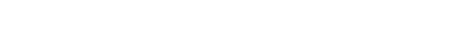3. the area of the region enclosed by the curve y = 4 − x2, the line y = 3x and the y-axis (2marks)5. The positions of two towns A and B are (500N, 450W) and (500N, K0W) respectively. It takes a plane 5 hours to travel from A to B at an average speed of 800knots. The same plane takes 1½ hours to travel from B to another town C at the same average speed. Given that C is to the north of B, calculate to the nearest degree,
1. The value of K (4marks)D=S×T
=800×5
=4000nm
1° = 60Cos50°
?      4000
4000 × 1
60Cos50°
= 103.7°
K − 45 = 103.7
K = 103.7 + 45
= 148.7°W
2. The latitude of C (3marks)
D = S × T
= 800 × 3/2 = 1200nm
1° = 60nm
?    1200nm
1200 × 1 =20°
60
x − 50 = 20
x = 70°W
3. If the plane started from A at 9.00am and flew to C through B, find the local time at C when the plane arrived there. (3marks)Distance A − B − C
= 4000nm + 1200
= 5200nm
T = D/S
= 5200
800
= 6½hrs
θ = 103.7°
1° = 4min
103.7 = 103.7 × 4 = 414.8 = 6hrs 55min
60
9:00
−6:55
02:05a.m
+6:30
8:35a.m at C
6.
1. Complete the table below for the equation y = x3 + 2x2 to 2 d.p(2marks)
 x −3 −2.5 −2 −1.5 −1 −0.5 0 0.5 1 1.5 2x2 18 12.5 8 4.5 2 0.5 0 0.5 1 4.5 x3 −27 −15.63 −8 −3.38 −1 −0.13 0 0.13 1 3.38 y −9 −3.13 0 1.12 1 0.37 0 0.63 2 7.88
2. On the grid provided, draw the graph of y = x3 + 2x2 for −3 ≤ x ≤ 1.5. Take a scale of 2cm to represent 1 unit on the x- axis and 1cm to represent 1unit on the y – axis. (3marks)3. Use your graph to solve
1. x3 + 2x2 = 0        (2marks)
2. x3 + 2x2 − x − 2 = 0      (3marks)
7. The table below shows the marks obtained by 47 students in a mathematics test.
 Marks 31 – 35 36 – 40 41 – 45 46 – 50 51 – 55 56 - 60 No. of candidates 4    4 6   10 12    22 15    37 8    45 2    47
1. On the grid provided, draw a cumulative frequency curve. (3marks)1. The median (2marks)
47/2 = 23.5    46
2. The semi interquartile range (2marks)
2. In order to pass the test a candidate had to score more than 40 marks. Calculate the percentage of candidates who passed. (3marks)
8. In the triangle OPQ below, OP = p and OQ = q. R is a point on PQ such that PR: RQ = 1 : 3 and 5OS = 2 OQ. PS intersects OR at T.1. Express in term of p and q
1. OS (1mark)
→
OS = 2/5 OQ  = 2/5q
2. PQ (1mark)
PQ = qp
3. OR (2mark)
OR = ¼q + ¾p
2. Given that OT= hOR and PT = kPS. Determine the values of h and k. (6marks)
1.      →        →
OT = h OR
= h(¼q + ¾p)
= h/4q + 3h/4p
2. →      →     →
OT = OP + PT
= p + k (−p + 2/5q)
= p − kp + 2k/5q
= p(1−k) + 2k/5q
h/4q + 3h/4p = p(1−k) + 2k/5q
3h/4 = 1 − k
h/4 = 2k/5
h = 8/5k
¾(8/5k) = 1− k
6/5k + 5/5k = 1
11/5k = 1
k = 5/11
h = 8/5(5/11)
h = 8/11

• ✔ To read offline at any time.
• ✔ To Print at your convenience
• ✔ Share Easily with Friends / Students